# HashMap实现原理

0收藏

### 前言

HashMap的主干是一个数组，假设我们有3个键值对dnf:1，cf:2，lol:3，每次放的时候会根据hash函数来确定这个键值对应该放在数组的哪个位置，即index = hash(key)

1 = hash(dnf)，我们将键值对放在数组下标为1的位置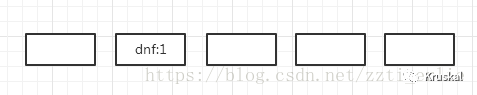3 = hash(cf)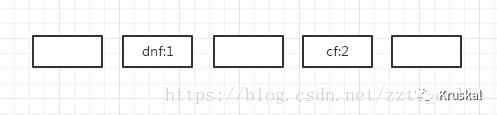1 = hash(lol)，这时发现数组下标为1的位置已经有值了，我们就可以用链表的形式将这个键值对放到dnf键值对的下面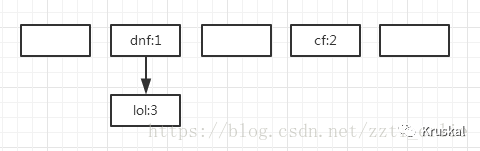### 源码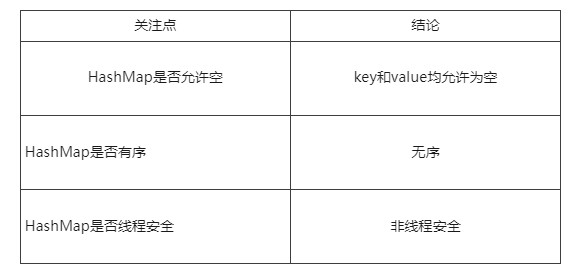``````//放置的key-value对的个数
transient int size;

//HashMap的主干是一个Entry数组,在需要的时候进行扩容，长度必须是2的被数
transient Entry<K,V>[] table = (Entry<K,V>[]) EMPTY_TABLE;

//进行扩容的阀值，值为 capacity * load factor，即容量 * 负载因子
int threshold;

//负载因子

threshold = capacity * load factor，即扩容的阀值=容量*负载因子，

1.负载因子越小，容易扩容，浪费空间，但查找效率高

2.负载因子越大，不易扩容，对空间的利用更加充分，查找效率低（链表拉长）

``````static class Entry<K,V> implements Map.Entry<K,V> {
final K key;
V value;
Entry<K,V> next;//存储指向下一个Entry的引用，单链表结构
int hash;//对key的hashcode值进行hash运算后得到的值，存储在Entry，避免重复计算

Entry(int h, K k, V v, Entry<K,V> n) {
value = v;
next = n;
key = k;
hash = h;
}
}``````

``````public HashMap(int initialCapacity, float loadFactor) {
threshold = initialCapacity;
}``````

put操作

``````public V put(K key, V value) {
//hashmap的数组={}
if (table == EMPTY_TABLE) {
inflateTable(threshold);
}
if (key == null)
return putForNullKey(value);
//获取hash值
int hash = hash(key);
//找到应该放到table的哪个位置
int i = indexFor(hash, table.length);
//遍历table[i]位置的链表，查找相同的key,若找到则使用新的value替换oldValue,并返回oldValue
for (Entry<K,V> e = table[i]; e != null; e = e.next) {
Object k;
//如果key已经存在，将value设置为新的，并返回旧的value值
if (e.hash == hash && ((k = e.key) == key || key.equals(k))) {
V oldValue = e.value;
e.value = value;
e.recordAccess(this);
return oldValue;
}
}

modCount++;
//将元素放到table[i]，新的元素总在table[i]位置的第一个元素，原来的元素后移
return null;
}``````

``````private void inflateTable(int toSize) {
//返回大于或等于最接近2的幂数
int capacity = roundUpToPowerOf2(toSize);

threshold = (int) Math.min(capacity * loadFactor, MAXIMUM_CAPACITY + 1);
table = new Entry[capacity];
initHashSeedAsNeeded(capacity);
}``````

``````private V putForNullKey(V value) {
for (Entry<K,V> e = table; e != null; e = e.next) {
if (e.key == null) {
V oldValue = e.value;
e.value = value;
e.recordAccess(this);
return oldValue;
}
}
modCount++;
return null;
}``````

``````void addEntry(int hash, K key, V value, int bucketIndex) {
//容量超过阈值，并且即将发生哈希冲突时进行扩容
if ((size >= threshold) && (null != table[bucketIndex])) {
//扩容为原来的2倍
resize(2 * table.length);
//重新计算hash值
hash = (null != key) ? hash(key) : 0;
bucketIndex = indexFor(hash, table.length);
}

createEntry(hash, key, value, bucketIndex);
}``````

``````void createEntry(int hash, K key, V value, int bucketIndex) {
Entry<K,V> e = table[bucketIndex];
table[bucketIndex] = new Entry<>(hash, key, value, e);
size++;
}``````

get方法

``````public V get(Object key) {
if (key == null)
return getForNullKey();
Entry<K,V> entry = getEntry(key);

return null == entry ? null : entry.getValue();
}``````

``````private V getForNullKey() {
if (size == 0) {
return null;
}
for (Entry<K,V> e = table; e != null; e = e.next) {
if (e.key == null)
return e.value;
}
return null;
}``````

key不为null时

``````final Entry<K,V> getEntry(Object key) {
if (size == 0) {
return null;
}

int hash = (key == null) ? 0 : hash(key);
for (Entry<K,V> e = table[indexFor(hash, table.length)];
e != null;
e = e.next) {
Object k;
if (e.hash == hash &&
((k = e.key) == key || (key != null && key.equals(k))))
return e;
}
return null;
}``````

### HashMap的大小为什么是2的倍数

h是hashcode，length时数组长度，下面这个方法是根据hashcode求出对象在数组中放置的位置

``````static int indexFor(int h, int length) {
return h & (length-1);
}``````

h & (length - 1) 等价于 h % length，我们假设数组的长度为15和16，hashcide为8和9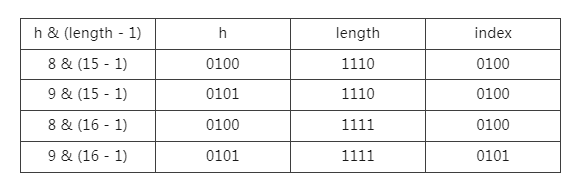帖子
视频
声望
粉丝
最近发布
社区精华内容